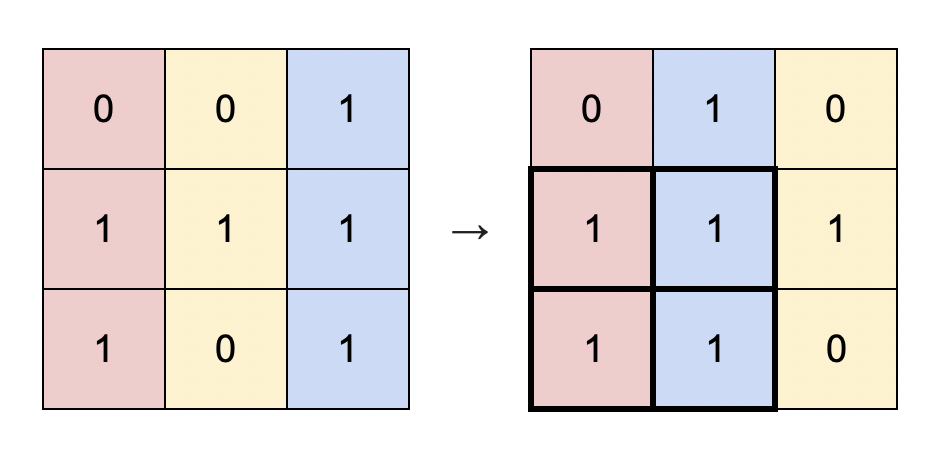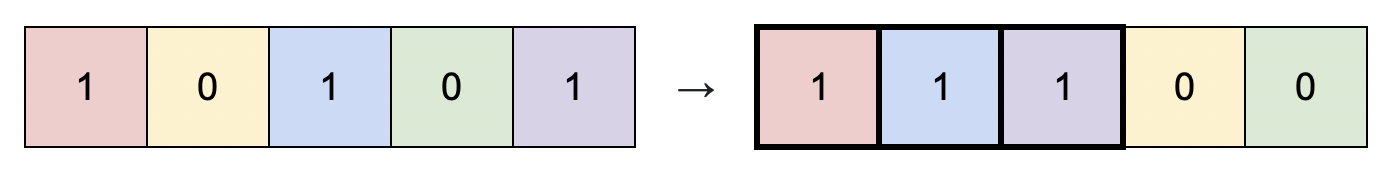# 重新排列后的最大子矩阵

## 1727. 重新排列后的最大子矩阵 (Medium)```输入：matrix = [[0,0,1],[1,1,1],[1,0,1]]

``````输入：matrix = [[1,0,1,0,1]]

```

```输入：matrix = [[1,1,0],[1,0,1]]

```输入：matrix = [[0,0],[0,0]]

• `m == matrix.length`
• `n == matrix[i].length`
• `1 <= m * n <= 105`
• `matrix[i][j]` 要么是 `0` ，要么是 `1`

### 相关话题

[贪心] [数组] [矩阵] [排序]